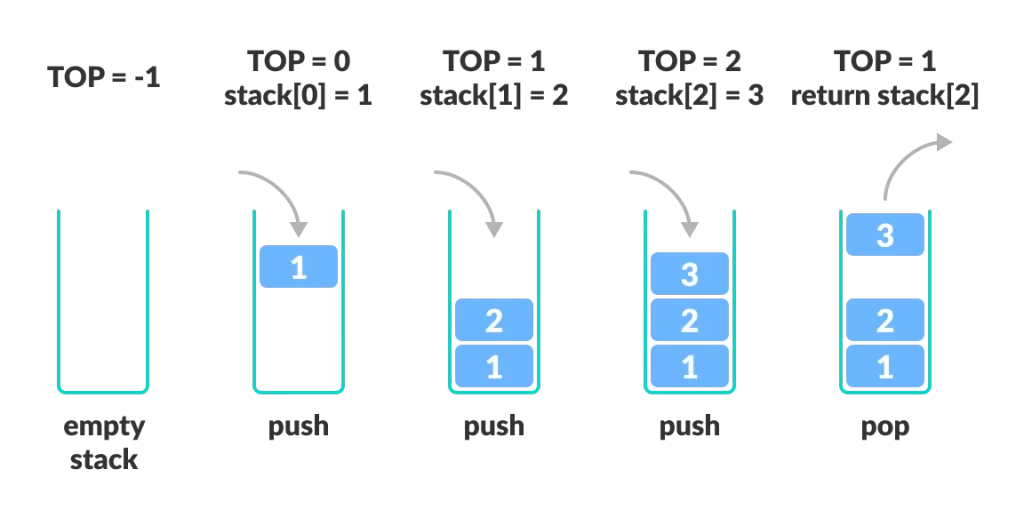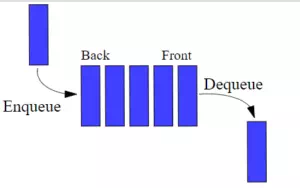# How To Use Python Lists To Implement Stack And Queue In Python Example

Python lists are versatile data structures that can be used to implement stacks and queues efficiently. In this article, we will explore how to use Python lists to implement these fundamental data structures, along with examples illustrating their usage.

## 1. Implementing Stacks with Python Lists.

1. A stack is a linear data structure that follows the Last-In-First-Out (LIFO) principle.
2. In other words, the last element added to the stack is the first one to be removed.3. Python lists are well-suited for implementing stacks due to their append and pop operations, which can mimic the behavior of a stack.

### 1.1 Creating a Stack.

1. Let’s start by creating a basic stack class using a Python list.
2. Class Stack source code.
```class Stack:
def __init__(self):
self.items = []

def push(self, item):
self.items.append(item)

def pop(self):
if not self.is_empty():
return self.items.pop()

def is_empty(self):
return len(self.items) == 0

def peek(self):
if not self.is_empty():
return self.items[-1]

def size(self):
return len(self.items)
```

### 1.2 Example Usage of Stacks.

1. Now that we have our `Stack` class defined, let’s demonstrate how to use it.
```if __name__ == "__main__":
# Create a new stack
stack = Stack()

# Push elements onto the stack
stack.push(10)
stack.push(20)
stack.push(30)

print("stack.items: ", stack.items)

# Check the top element
print("Top element:", stack.peek())

# Pop elements from the stack
print("Popped:", stack.pop())
print("Popped:", stack.pop())

# Check if the stack is empty
print("Is the stack empty?", stack.is_empty())

# Get the size of the stack
print("Stack size:", stack.size())```
2. Output.

```stack.items:  [10, 20, 30]
Top element: 30
Popped: 30
Popped: 20
Is the stack empty? False
Stack size: 1```

## 2. Implementing Queues with Python Lists.

1. A queue is another linear data structure that follows the First-In-First-Out (FIFO) principle.2. Python lists can be adapted to implement queues efficiently.

### 2.1 Creating a Queue.

1. Here’s a basic queue class using a Python list.
2. Class Queue source code.
```class Queue:
def __init__(self):
self.items = []

def enqueue(self, item):
self.items.insert(0, item)
print('self.items: ', self.items)

def dequeue(self):
if not self.is_empty():
return self.items.pop()

def is_empty(self):
return len(self.items) == 0

def size(self):
return len(self.items)```

### 2.2 Example Usage of Queues.

1. Now, let’s see how to use the `Queue` class.
```if __name__ == "__main__":
# Create a new queue
queue = Queue()

# Enqueue elements
queue.enqueue(10)
queue.enqueue(20)
queue.enqueue(30)

print('queue.items: ', queue.items)

# Dequeue elements
print("Dequeued:", queue.dequeue())
print("Dequeued:", queue.dequeue())

# Check if the queue is empty
print("Is the queue empty?", queue.is_empty())

# Get the size of the queue
print("Queue size:", queue.size())```
2. Output.

```self.items:  
self.items:  [20, 10]
self.items:  [30, 20, 10]
queue.items:  [30, 20, 10]
Dequeued: 10
Dequeued: 20
Is the queue empty? False
Queue size: 1```

## 3. Conclusion.

1. Python lists are versatile and can be used to implement stacks and queues efficiently.
2. By using appropriate methods such as `append` and `pop` for stacks, and `insert` and `pop` for queues, you can create these essential data structures to manage and manipulate your data effectively.
3. Understanding how to implement stacks and queues using Python lists is valuable for solving various real-world problems and optimizing your code.

This site uses Akismet to reduce spam. Learn how your comment data is processed.